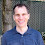## Sunday, June 30, 2019

### A proof that 22/7 - pi > 0 and more

My father was a High School English teacher who did not know much math. As I was going off to college, intending to major in math, he gave me the following sage advice:

1) Take Physics as well as Math since Physics and Math go well together. This was good advice. I took the first year of Physics for Physics Majors, and I later took a senior course in Mechanics since that was my favorite part of the first year course. Kudos to Dad!

2) π is exactly 22/7. I knew this was not true, but I also knew that I had no easy way to show him this. In fact, I wonder if I could have proven it myself back then.

Problem A-1 on the 1968 Putnam exam:

Prove 22/7 - π = ∫01 (x4(1-x)4)/(1+ x2 )dx

(I can easily do his by partial fractions and remembering that ∫ 1/(1+x^2) dx = tan-1x which is tan inverse, not 1/tan. See here.)

(ADDED LATER---I have added conjectures on getting integrals of the form above except with 4 replaced by any natural number. Be the first on your block to solve my conjectures! It has to be easier than the Sensitivity Conjecture!)

Let n ∈ N which we will choose later. By looking at the circle that is inscribed in a regular n-polygon (n even) one finds that

n tan(π/n) > π

So we seek an even value of n such that

n tan(π/n) < 22/7

Using Wolfram alpha the smallest such n is 92.

Would that convince Dad? Would he understand it? Probably not. Oh well.

Some misc points.

1) While working on this post I originally wanted to find tan(π/27) by using the half-angle formula many times, and get an exact answer in terms of radicals, rather than using Wolfram Alpha.

a) While I have lots of combinatorics books, theory of comp books, and more Ramsey Theory books than one person should own in my house, I didn't have a SINGLE book with any trig in it.

b) I easily found it on the web:

tan(x/2) = sqrt( (1-cos x)/(1+cos x) ) = sin x/(1+cos x) = (1-cos x)/(sin x).

None of these seems like it would get me a nice expression for tan(π/27). But I don't know. Is there a nice expression for tan(π/2k) ? If you know of one then leave a polite comment.

2) I assumed that there was a more clever and faster way to do the integral. I could not find old Putnam exams and their solutions the web (I'm sure they are there someplace! --- if you know then comment politely with a pointer). So I got a book out of the library The William Lowell Putnam Mathematical Competition Problems and Solutions 1965--1984 by Alexanderson, Klosinski, and Larson. Here is the clever solution:

The standard approach from Elementary Calculus applies.

Not as clever as I as hoping for.

3) I also looked at the integral with 4 replaced by 1,2,3,4,...,16. The results are in the writeup I pointed to before. It looks like I can use this sequence to get upper and lower bound on pi, ln(2), pi+2ln(2), and pi-2ln(2). I have not proven any of this. But take a look! And as noted above I have conjectures!

4) When I looked up INSCRIBING a circle in a regular n-polygon, Google kept giving me CIRCUMSCRIBING. Why? I do not know but I can speculate. Archimedes had a very nice way of using circumscribed circles to approximate pi. Its on youtube here. Hence people are used to using circumscribed rather than inscribed circles.

1.Here is a link to a solution of the Putnam problem:
http://mks.mff.cuni.cz/kalva/putnam/putn68.html

2.3.I'd avoid W Alpha or W Beta. Both mentioning and using.

4.I got email from someone saying:

Just type 22/7 - pi into Wolfram Alpha.

while funny it raises a good point- would that convince my dad? Should it convince you? Note that my lack of thinking to do that lead to this post and some interesting ways to approx pi, ln(2),
pi-2ln(2) and pi+2ln(2).

5.According to the book "The Historical Development of the Calculus" by C.H. Edwards, Jr., 1979, Chapter 2, Archimedes used inscribed and circumscribed polygons to get a very tight bound on the value of pi. See the book for details.

6.1.